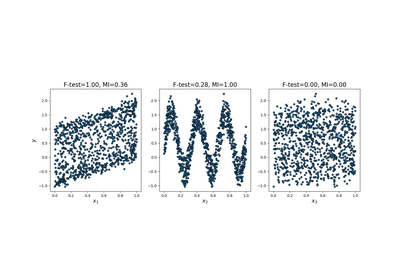# `sklearn.feature_selection`.mutual_info_regression¶

`sklearn.feature_selection.``mutual_info_regression`(X, y, discrete_features=’auto’, n_neighbors=3, copy=True, random_state=None)[source]

Estimate mutual information for a continuous target variable.

Mutual information (MI) [R176] between two random variables is a non-negative value, which measures the dependency between the variables. It is equal to zero if and only if two random variables are independent, and higher values mean higher dependency.

The function relies on nonparametric methods based on entropy estimation from k-nearest neighbors distances as described in [R177] and [R178]. Both methods are based on the idea originally proposed in [R179].

It can be used for univariate features selection, read more in the User Guide.

Parameters: X : array_like or sparse matrix, shape (n_samples, n_features) Feature matrix. y : array_like, shape (n_samples,) Target vector. discrete_features : {‘auto’, bool, array_like}, default ‘auto’ If bool, then determines whether to consider all features discrete or continuous. If array, then it should be either a boolean mask with shape (n_features,) or array with indices of discrete features. If ‘auto’, it is assigned to False for dense X and to True for sparse X. n_neighbors : int, default 3 Number of neighbors to use for MI estimation for continuous variables, see [R177] and [R178]. Higher values reduce variance of the estimation, but could introduce a bias. copy : bool, default True Whether to make a copy of the given data. If set to False, the initial data will be overwritten. random_state : int, RandomState instance or None, optional, default None The seed of the pseudo random number generator for adding small noise to continuous variables in order to remove repeated values. If int, random_state is the seed used by the random number generator; If RandomState instance, random_state is the random number generator; If None, the random number generator is the RandomState instance used by np.random. mi : ndarray, shape (n_features,) Estimated mutual information between each feature and the target.

Notes

1. The term “discrete features” is used instead of naming them “categorical”, because it describes the essence more accurately. For example, pixel intensities of an image are discrete features (but hardly categorical) and you will get better results if mark them as such. Also note, that treating a continuous variable as discrete and vice versa will usually give incorrect results, so be attentive about that.
2. True mutual information can’t be negative. If its estimate turns out to be negative, it is replaced by zero.

References

 [R176] (1, 2) Mutual Information on Wikipedia.
 [R177] (1, 2, 3) A. Kraskov, H. Stogbauer and P. Grassberger, “Estimating mutual information”. Phys. Rev. E 69, 2004.
 [R178] (1, 2, 3) B. C. Ross “Mutual Information between Discrete and Continuous Data Sets”. PLoS ONE 9(2), 2014.
 [R179] (1, 2) L. F. Kozachenko, N. N. Leonenko, “Sample Estimate of the Entropy of a Random Vector”, Probl. Peredachi Inf., 23:2 (1987), 9-16

## Examples using `sklearn.feature_selection.mutual_info_regression`¶Comparison of F-test and mutual information